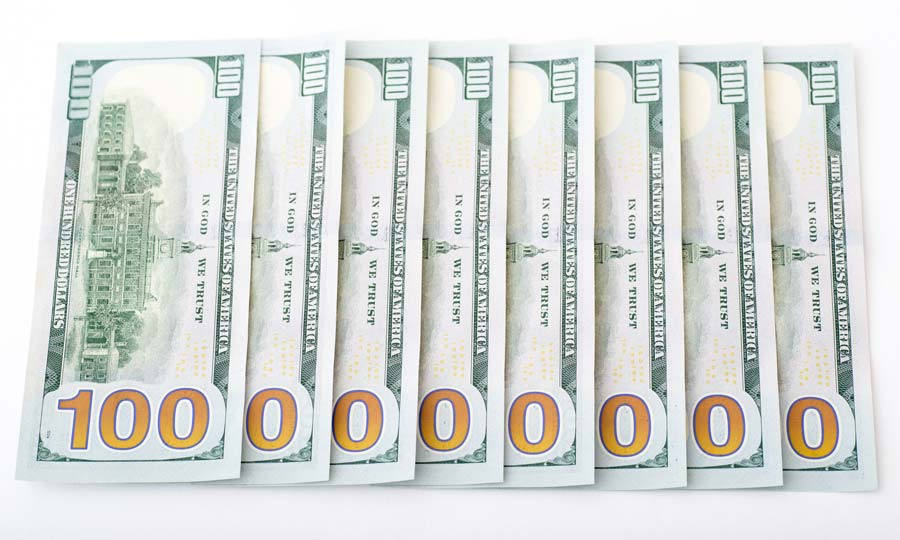# Million to Billion Converter

Use this calculator to convert millions to billions and billions to millions.

Disclaimer: Whilst every effort has been made in building our calculator tools, we are not to be held liable for any damages or monetary losses arising out of or in connection with their use. Full disclaimer.

## How many million are in one billion?

The meaning of a billion is one thousand million (1,000,000,000). This figure was principally declared as the billion in American English, and was then adopted as the common measurement for billion in British English in the 1970s.

1 billion = 1000 million

To give you some monetary context, if you were to earn \$100,000 per year it would take you 10 years to earn \$1 million. To reach \$1 billion would require you to save every penny you earn for 10,000 years.

## How to convert million to billion

1 million is equal to 0.001 billion. To convert your million figure to billion, divide it by 1000.

## How to convert billion to million

1 billion is equal to 1,000 million. To convert your billion figure to million, multiply it by 1000.

## How many zeros in 1 billion?

Well, like so many things when it comes to measuring up, it can vary a bit depending on where you are. In the US, a billion has always been < Dr. Evil > ONE THOUSAND MILLION < / Dr. Evil > . In numbers, that's 1,000,000,000 with, count 'em, nine zeroes.In the UK, traditionally a billion was < Dr. Evil second attempt > ONE MILLION MILLION < / second attempt > . That's 12 zeroes (1,000,000,000,000). But what's a difference of three zeroes between friends? A lot, as it happens.

A doctor telling you that you have a billion seconds to live would receive a stony reception in the US, as that adds up to 32 years. In the land of red phone boxes, cups of tea and roast beef, though, it would mean 32,000 years. Suddenly, British immortality is explained: Doctor Who regenerating across centuries, and Mick Jagger, looking youthful at just 240 years old - it all makes sense.

BUT WAIT!

In 1974, the UK government decided to try on a leather jacket and talk like the Fonz, and it began using the US sense of "billion" for all official figures released - that's a thousand million. Nine zeroes. It's basically a universal now. 1

A billion:

1,000,000,000

## Million to billion chart

Million to billion Million to billion
1 million = 0.001 billion20 million = 0.02 billion
2 million = 0.002 billion30 million = 0.03 billion
3 million = 0.003 billion40 million = 0.04 billion
4 million = 0.004 billion50 million = 0.05 billion
5 million = 0.005 billion60 million = 0.06 billion
6 million = 0.006 billion70 million = 0.07 billion
7 million = 0.007 billion80 million = 0.08 billion
8 million = 0.008 billion90 million = 0.09 billion
9 million = 0.009 billion100 million = 0.1 billion
10 million = 0.01 billion110 million = 0.11 billion

## Billion to million chart

Billion to million Billion to million
1 billion = 1,000 million11 billion = 11,000 million
2 billion = 2,000 million12 billion = 12,000 million
3 billion = 3,000 million13 billion = 13,000 million
4 billion = 4,000 million14 billion = 14,000 million
5 billion = 5,000 million15 billion = 15,000 million
6 billion = 6,000 million16 billion = 16,000 million
7 billion = 7,000 million17 billion = 17,000 million
8 billion = 8,000 million18 billion = 18,000 million
9 billion = 9,000 million19 billion = 19,000 million
10 billion = 10,000 million20 billion = 20,000 million

## What does a billion look like?

Some things are too extraordinary to visualise. Can you even imagine a billion dollars? A billion cats? A billion jars of Nutella, all with your name on them?

Let's go back to the idea that a billion seconds is 32 years. How many years, then, is a million seconds? Have a little think. The answer? None. It is no years. It is less than 12 days. That's how vast the difference is between million and billion.

Now raid your piggy bank and see if you can find a billion pennies or cents to pile up. That's 10 million dollars, pounds or euros, so it's probably not worth wasting your time actually smashing open Percy Pig right now. But just imagine: one billion cents in a stack would be 870 miles high. Or if you laid them side by side in a line, it would stretch 6,213 miles, which would reach 2/3 of the way round the circumference of Mercury.

We hope you've found this article and converter helpful. When you next hear someone talk about money in the billions, you'll know how much that equates to.

If you think the billion is a huge number, though, it's nothing compared to the size of the trillion.

Calculator: Alastair Hazell. Article: Becky Kleanthous.

### References

1. House of Commons Library. What is a billion? And other units.